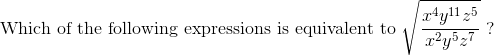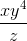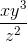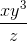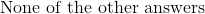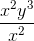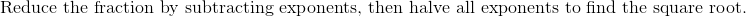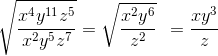# High School Math : Exponential and Logarithmic Functions

## Example Questions

### Example Question #1 : Exponential And Logarithmic Functions

You are given that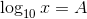and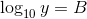Which of the following is equal to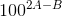?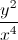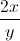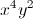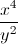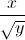Explanation:

Sinceand, it follows that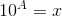and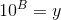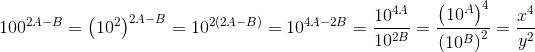### Example Question #1 : Solving Logarithms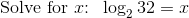Explanation: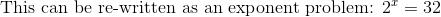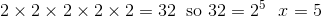### Example Question #3 : Exponential And Logarithmic Functions

What is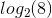Explanation:

Recall that by definition a logarithm is the inverse of the exponential function. Thus, our logarithm corresponds to the value ofin the equation: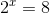We know that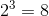and thus our answer is.

### Example Question #4 : Exponential And Logarithmic Functions

Solve for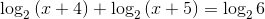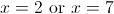The correct solution set is not included among the other choices.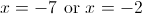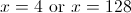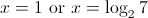The correct solution set is not included among the other choices.

Explanation: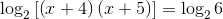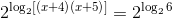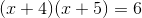FOIL: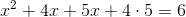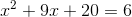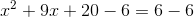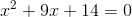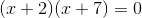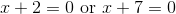These are our possible solutions. However, we need to test them.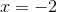: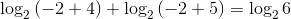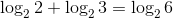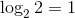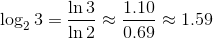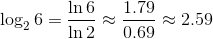The equation becomes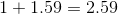. This is true, sois a solution.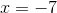: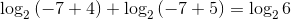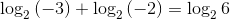However, negative numbers do not have logarithms, so this equation is meaningless.is not a solution, andis the one and only solution. Since this is not one of our choices, the correct response is "The correct solution set is not included among the other choices."

### Example Question #1 : Simplifying Exponential Functions